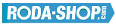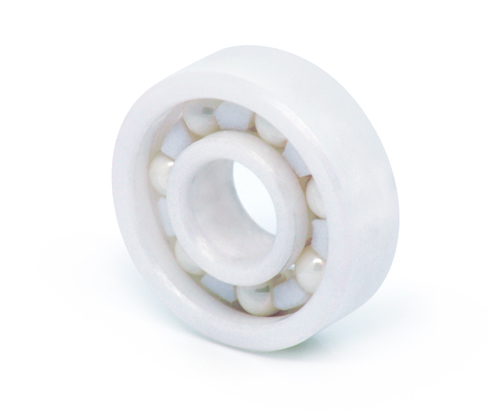# Hybrid and Ceramic BearingsManufacturer Product Image Item Name Price### 6206-CE-SI3N4-PFTE

Solid Ceramic Bearings Ceramic Open(No Shields) Dimension d=30 Dimension D=62 Dimension B=16 Dinamic Load Rating=11700 Static Load Rating=6780 Weight=0.199gr. Speed limit Grease=N/A Speed limit Oil=0 Flanged
inside diameter = 30
outside diameter = 62
width = 16### 6206-CE-ZR02-PFTE

Solid Ceramic Bearings Ceramic Open(No Shields) Dimension d=30 Dimension D=62 Dimension B=16 Dinamic Load Rating=11700 Static Load Rating=6780 Weight=0.199gr. Speed limit Grease=N/A Speed limit Oil=0 Flanged
inside diameter = 30
outside diameter = 62
width = 16
call### 6207

Solid Ceramic Bearings Ceramic Open(No Shields) Dimension d=35 Dimension D=72 Dimension B=17 Dinamic Load Rating=15420 Static Load Rating=9180 Weight=0.284gr. Speed limit Grease=N/A Speed limit Oil=0
inside diameter = 35
outside diameter = 72
width = 17### 6208

Solid Ceramic Bearings Ceramic Open(No Shields) Dimension d=40 Dimension D=80 Dimension B=18 Dinamic Load Rating=17460 Static Load Rating=10740 Weight=0.366gr. Speed limit Grease=N/A Speed limit Oil=0
inside diameter = 40
outside diameter = 80
width = 18### 628-CE-SI3N4-PFTE

Solid Ceramic Bearings Ceramic Open(No Shields) Dimension d=8 Dimension D=24 Dimension B=8 Dinamic Load Rating=2000 Static Load Rating=854 Weight=0.018gr. Speed limit Grease=N/A Speed limit Oil=0 Flanged
inside diameter = 8
outside diameter = 24
width = 8### 628-CE-ZR02-PFTE

Solid Ceramic Bearings Ceramic Open(No Shields) Dimension d=8 Dimension D=24 Dimension B=8 Dinamic Load Rating=2000 Static Load Rating=854 Weight=0.018gr. Speed limit Grease=N/A Speed limit Oil=0 Flanged
inside diameter = 8
outside diameter = 24
width = 8
call### 629

Solid Ceramic Bearings Ceramic Open(No Shields) Dimension d=9 Dimension D=26 Dimension B=8 Dinamic Load Rating=2745 Static Load Rating=1190 Weight=0.022gr. Speed limit Grease=N/A Speed limit Oil=0
inside diameter = 9
outside diameter = 26
width = 8### 6300-CE-SI3N4-PFTE

Solid Ceramic Bearings Ceramic Open(No Shields) Dimension d=10 Dimension D=35 Dimension B=11 Dinamic Load Rating=4860 Static Load Rating=2070 Weight=0.052gr. Speed limit Grease=N/A Speed limit Oil=0 Flanged
inside diameter = 10
outside diameter = 35
width = 11### 6300-CE-ZR02-PFTE

Solid Ceramic Bearings Ceramic Open(No Shields) Dimension d=10 Dimension D=35 Dimension B=11 Dinamic Load Rating=4860 Static Load Rating=2070 Weight=0.052gr. Speed limit Grease=N/A Speed limit Oil=0 Flanged
inside diameter = 10
outside diameter = 35
width = 11
call### 6301-CE-SI3N4-PFTE

Solid Ceramic Bearings Ceramic Open(No Shields) Dimension d=12 Dimension D=37 Dimension B=12 Dinamic Load Rating=5820 Static Load Rating=2520 Weight=0.06gr. Speed limit Grease=N/A Speed limit Oil=0 Flanged
inside diameter = 12
outside diameter = 37
width = 12